Next: Worked example 5.2: Dragging Up: Conservation of energy Previous: Power

## Worked example 5.1: Bucket lifted from a well

Question: A man lifts a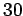kg bucket from a well whose depth is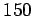m. Assuming that the man lifts the bucket at a constant rate, how much work does he perform?

Answer: Letbe the mass of the bucket and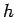the depth of the well. The gravitational force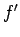acting on the bucket is of magnitude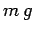and is directed vertically downwards. Hence,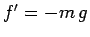(where upward is defined to be positive). The net upward displacement of the bucket is. Hence, the work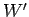performed by the gravitational force is the product of the (constant) force and the displacement of the bucket along the line of action of that force: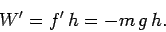Note thatis negative, which implies that the gravitational field surrounding the bucket gains energy as the bucket is lifted. In order to lift the bucket at a constant rate, the man must exert a forceon the bucket which balances (and very slightly exceeds) the force due to gravity. Hence,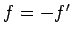. It follows that the work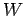done by the man is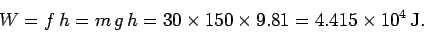Note that the work is positive, which implies that the man expends energy whilst lifting the bucket. Of course, since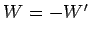, the energy expended by the man equals the energy gained by the gravitational field.Next: Worked example 5.2: Dragging Up: Conservation of energy Previous: Power
Richard Fitzpatrick 2006-02-02